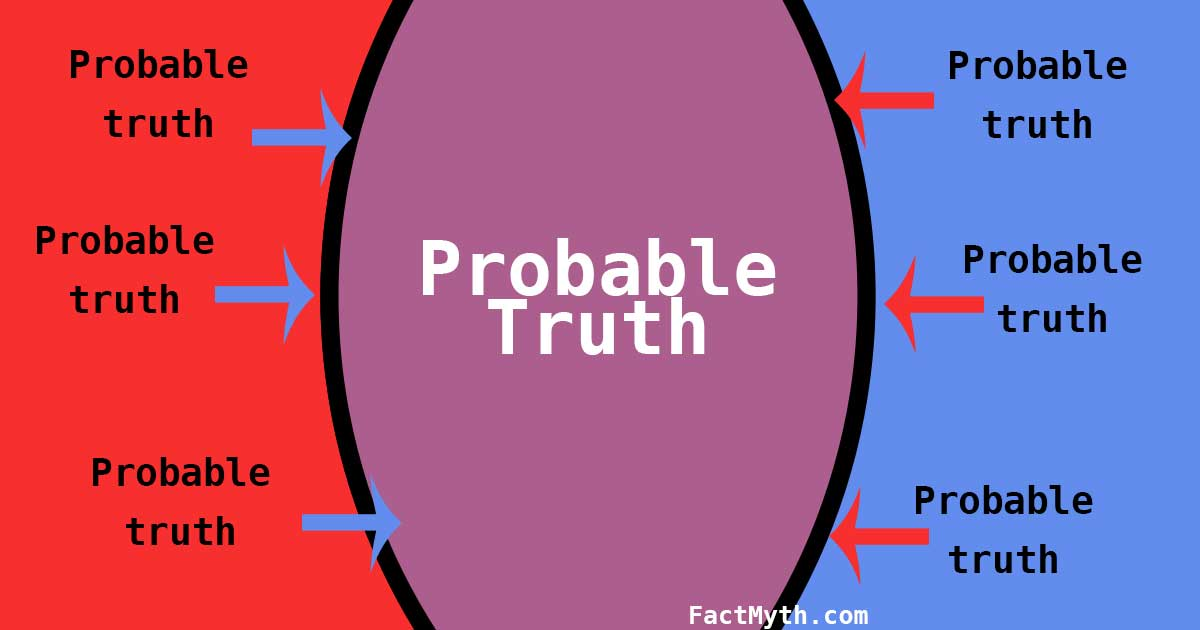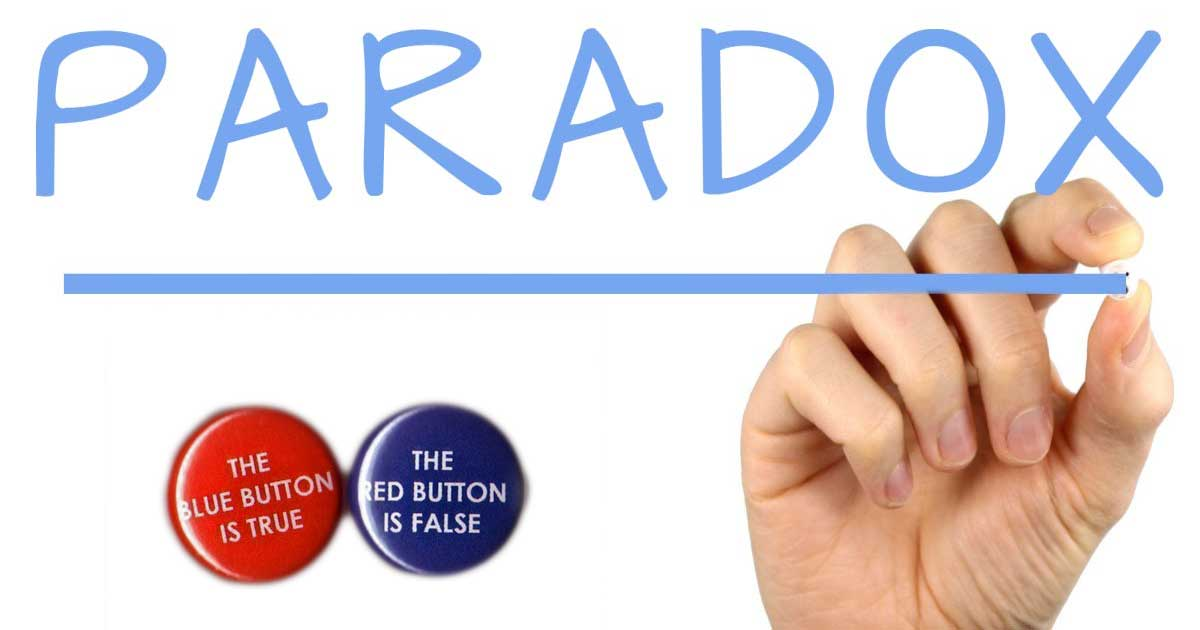Probability is a measure of the likeliness that an event will occur. Odds are a way of expressing probability.

Typically probability refers to the likely-hood or chance that something will happen. However, we can also measure the odds or probability that something won’t occur. Odds and probability are always a theoretical measure of chance as opposed to a guarantee that something will happen. Probability and odds are calculated mathematically, but not all factors related to a final equation are rooted in mathematics (such as human behavior, legislation, and changes in weather patterns).

Probability and odds are vital to fields of statistics, mathematics, finance, gambling, science, artificial intelligence, computing, game theory, philosophy, healthcare, security, weather, big data, and more. When people think of odds, they may think of “the odds of winning the lotto”, but the statistics based skill-set behind probability and odds have far reaching applications.

How to Express Probability Statistics: In statistics the probability (or “chance”) of rolling a 6 on a six sided die can be expressed as 1 in 6, 1/6, or 16.7%.

How to Express Odds in Statistics: The odds-for can be expressed as 1 to 5 (we expect 1 six event for ever 5 non-six events), and likewise the odds-against can be expressed as 5 to 1.

How to Express Odds in Gambling: In gambling odds can mean “payout” or probability. So in gambling 5 to 1 either means it pays \$5 for every \$1 bet. Or it means a 5 in 1 chance of happening (just like we express probability in statistics).

In every day language odds and probability are both used interchangeably to refer to “probability” or “chance”.

## Inductive Reasoning ExplainedWe explain inductive reasoning, a bottom-up reasoning method that reasons by consistency, comparing particulars and probabilities to find likely truths.

## What is a Truth-Value?A truth-value is a label that is given to a statement (a proposition) that denotes the relation of the statement to truth.